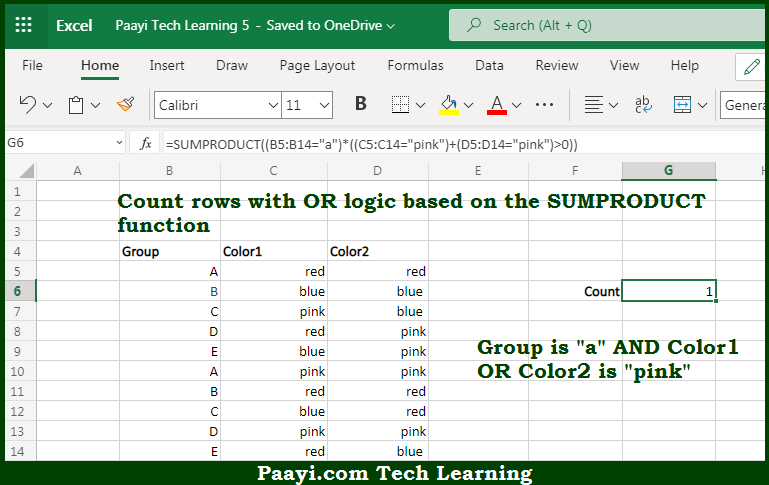# Learn How to COUNT Rows With OR Logic in Microsoft Excel

Written by | 0 Comments | 531 Views

In this article, you will learn how to COUNT various things in Microsoft Excel using a single or combination of functions and its purpose. You will also get to know how to COUNT Rows With OR Logic and see the generic formula.

COUNT Rows OR Logic in Microsoft Excel

The main purpose of this formula is to count rows with OR logic. Here we will learn how to count the rows using OR criteria in Microsoft Excel. That implies, with the help of a formula based on the SUMPRODUCT function you can able to count rows using OR logic. So, with the help of this formula, you can able to count rows using OR logic.

General Formula to COUNT Rows OR Logic

=SUMPRODUCT((group="a")*((color1="red")+(color2="red")>0))

The Explanation for the COUNT Rows OR LogicSo we know that with the help of the given formula above you can able to count rows with OR logic. Here we will learn how to count the rows using OR criteria in Microsoft Excel. As we know that one of the trickier issues in Microsoft Excel is to count rows in a set of data using OR logic. There are two basic situations, first, you want to count rows where a value in a column is "x" OR "y" and secondly you want to count rows where a value, "x", exists in one column OR another. In the example given above, the goal is to count rows. That implies we are working with the second scenario here. So, with the help of this formula, you can able to count rows using multiple criteria across different columns.